# 射频功率放大器 PA 的基本原理和信号分析

30 篇文章 4 订阅

RFPA 在射频发射机中的作用射频发射机主要包括信号源产生单元，调制以及功率放大等模块，中间再加入基本的滤波环节。对于一个功率放大器而言，其重要作用是扩大功率，获得足够的信号强度以便通过天线辐射出去；同时该过程信号不失真。因为无线WLAN信号是非恒定包络，它必须用线性放大器放大，才能满足信号不失真放大。同时802.11x所用频段ISM为公用频段，能提供的功率很小，一般小于1W（不同国家要求不同）。在这样的情况下，选择效率不高的A类功率放大器，能很好获得较优线性度。另一方面，由于A类放大器不需要额外的滤波电路，其封装可以很小，并且成本较低。u C E ≈ V c c ⋅ i c ⋅ R L u_{CE}≈V_{cc}\cdot i_{c}\cdot R_{L}
U B E U_{BE} 变小直至接近 U B E ( O N ) U_{BE(ON)} 时，即为B类功率放大器。

4.1 非线性特性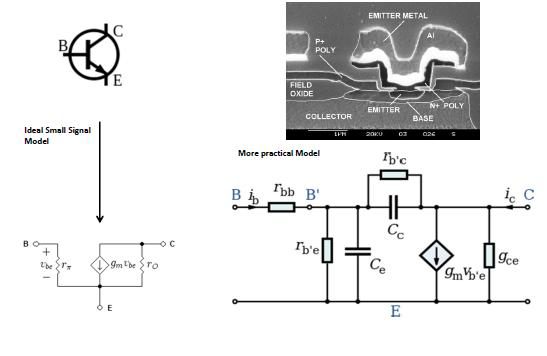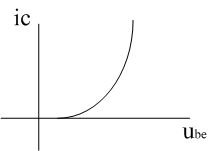i C ≈ I E B S e u b e U T i_{C}≈I_{EBS}e^{\frac{u_{be}}{U_{T}}}

y ( t ) = a 1 ⋅ x ( t ) + a 2 ⋅ x ( t ) 2 + a 3 ⋅ x ( t ) 3 + ⋅ ⋅ ⋅ y(t)=a_{1}\cdot x(t)+a_{2}\cdot x(t)^{2}+a_{3}\cdot x(t)^{3}+\cdot \cdot \cdot

4.2 非线性特性的影响

4.2.1 输入端仅有一个信号

y ( t ) = a 1 ⋅ A ⋅ c o s ( w t ) + a 2 ⋅ A 2 ⋅ c o s ( w t ) 2 + a 3 ⋅ A 3 ⋅ c o s ( w t ) 3 + ⋅ ⋅ ⋅ y(t)=a_{1}\cdot A\cdot cos(wt)+a_{2}\cdot A^{2}\cdot cos(wt)^{2}+a_{3}\cdot A^{3}\cdot cos(wt)^{3}+\cdot \cdot \cdot

= a 2 ⋅ A 2 / 2 + ( a 1 ⋅ A + 3 / 4 ⋅ a 3 ⋅ A 3 ) c o s ( w t ) + a 2 ⋅ A 2 / 2 ⋅ c o s ( 2 w t ) + a 3 ⋅ A 3 / 4 ⋅ c o s ( 3 w t ) + ⋅ ⋅ ⋅ =a_{2}\cdot A^{2}/2+(a_{1}\cdot A+3/4\cdot a_{3}\cdot A^{3})cos(wt)+a_{2}\cdot A^{2}/2\cdot cos(2wt)+a_{3}\cdot A^{3}/4\cdot cos(3wt)+\cdot \cdot \cdot (公式2)

y 1 ( t ) = ( a 1 ⋅ A + 3 / 4 ⋅ a 3 ⋅ A 3 ) c o s ( w t ) y_{1}(t)=(a_{1}\cdot A+3/4\cdot a_{3}\cdot A^{3})cos(wt) (公式3)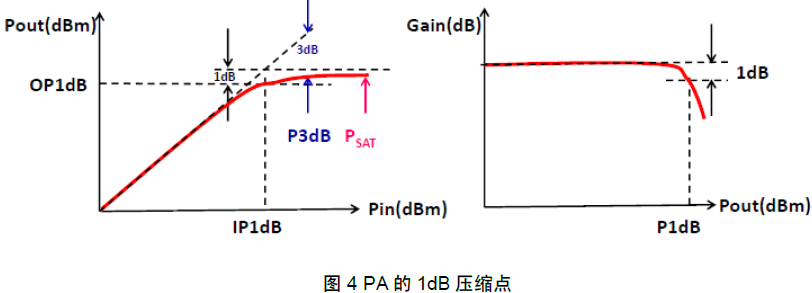20 ⋅ l o g ( a 1 + 3 / 4 ⋅ a 3 ⋅ A − 1 d B 2 ) = 20 ⋅ l o g ( a 1 ) − 1 d B 20\cdot log(a_{1}+3/4\cdot a_{3}\cdot A_{-1dB}^{2})=20\cdot log(a_{1})-1dB
A − 1 d B = 0.145 ⋅ ∣ a 1 / a 3 ∣ A_{-1dB}=\sqrt{0.145\cdot \left | a1 /a3\right |}
4.2.2 输入端有两个信号

x ( t ) = A 1 ∗ c o s ( ω 1 t ) + A 2 ∗ c o s ( ω 2 t ) x(t)=A_{1}*cos(ω_{1}t)+A_{2}*cos(ω_{2}t)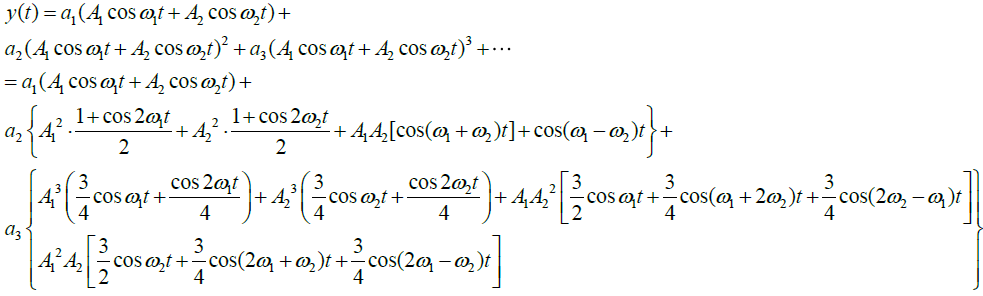由上式 可知，ω1 和ω2 的基频分量由一次方和三次方项产生：
y 1 （ t ） = ( a 1 A 1 + 3 / 4 ⋅ a 3 A 1 3 + 3 / 2 ⋅ a 3 A 1 A 2 2 ) c o s ω 1 t + ( a 1 A 2 + 3 / 4 ⋅ a 3 A 2 3 + 3 / 2 ⋅ a 3 A 2 A 1 2 ) y_{1}（t）=(a_{1}A_{1}+3/4\cdot a_{3}A_{1}^{3}+3/2\cdot a_{3}A_{1}A_{2}^{2})cosω_{1}t+(a_{1}A_{2}+3/4\cdot a_{3}A_{2}^{3}+3/2\cdot a_{3}A_{2}A_{1}^{2})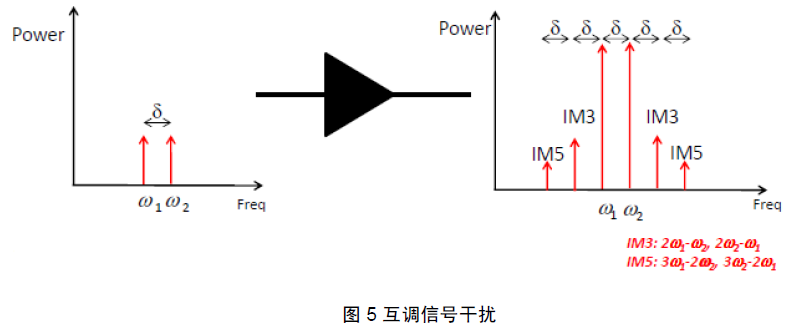a 1 A I P 3 3 = 3 / 4 ⋅ a 3 ⋅ A I P 3 3 a_{1}A_{IP3}^{3}=3/4\cdot a_{3}\cdot A_{IP3}^{3}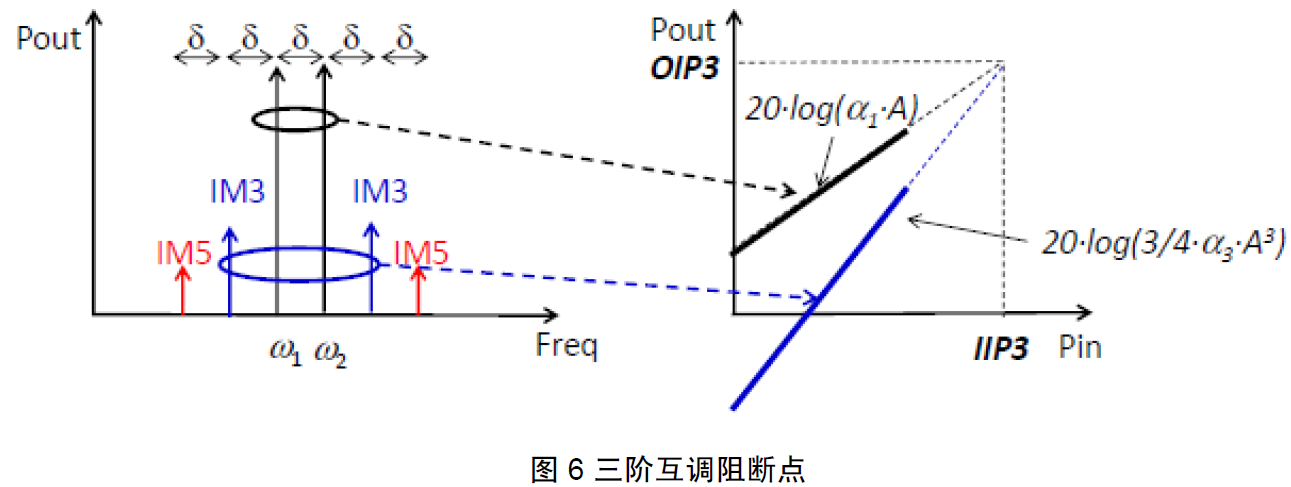4.2.3 边带信号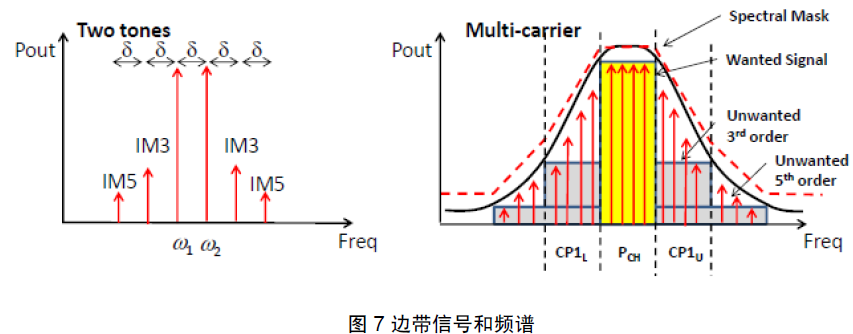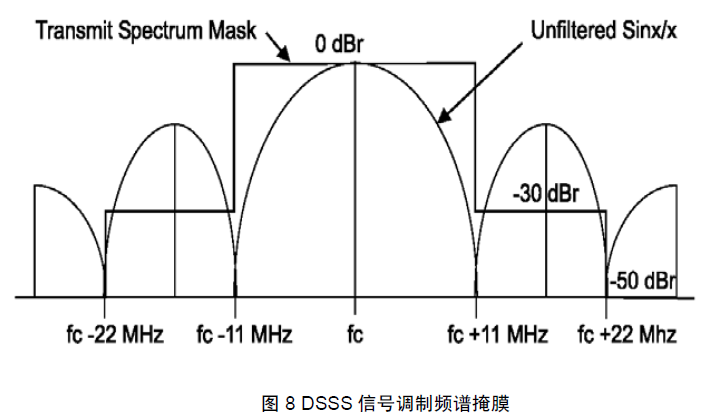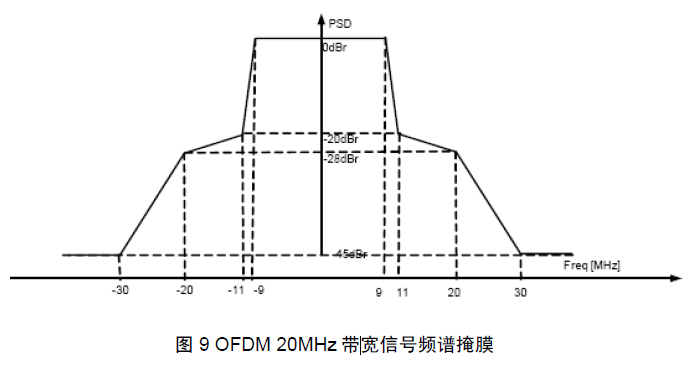4.2.4 非线性其他影响

08-0909-11825
01-043556
04-229111
11-212357
03-12707
11-071990
05-07
03-121755
02-28
01-078562
06-02
09-02点击重新获取扫码支付余额充值